并查集学习笔记

$\Huge\text{并查集学习笔记}$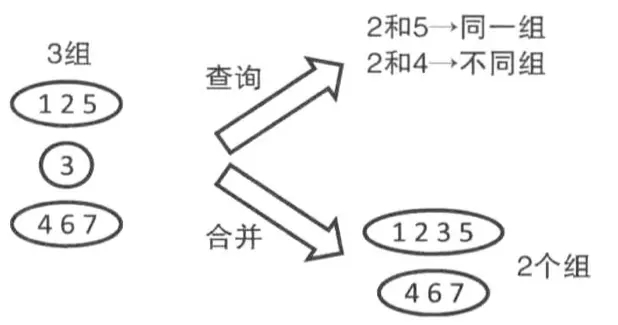1.查询$\text{find(x)}$（查询$x$所在集合的代表元素）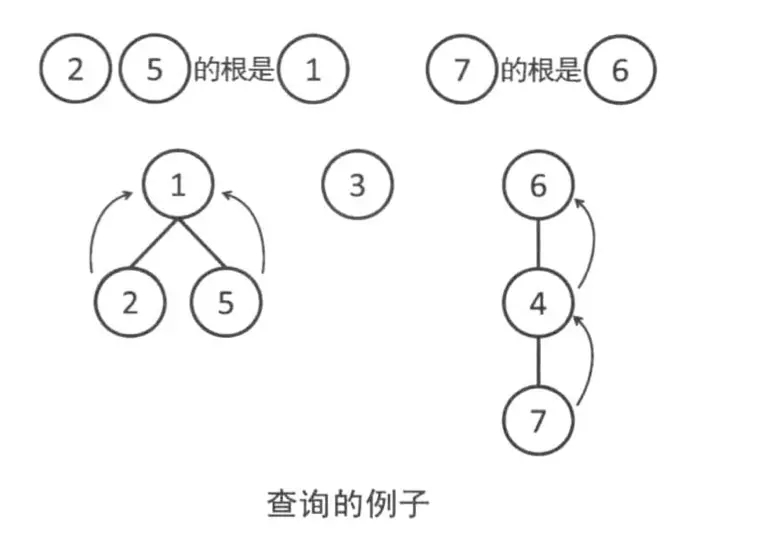int find (int x){
return fa[x]==x?x:find(fa[x]);
}

$fa$数组用于存储$x$元素所在集合的代表元素。在一开始每个元素的都是单独的所以$\text{fa[x]=x}$
2合并$\text{Union(x,y)}$（将$x$所在的集合与$y$所在的集合）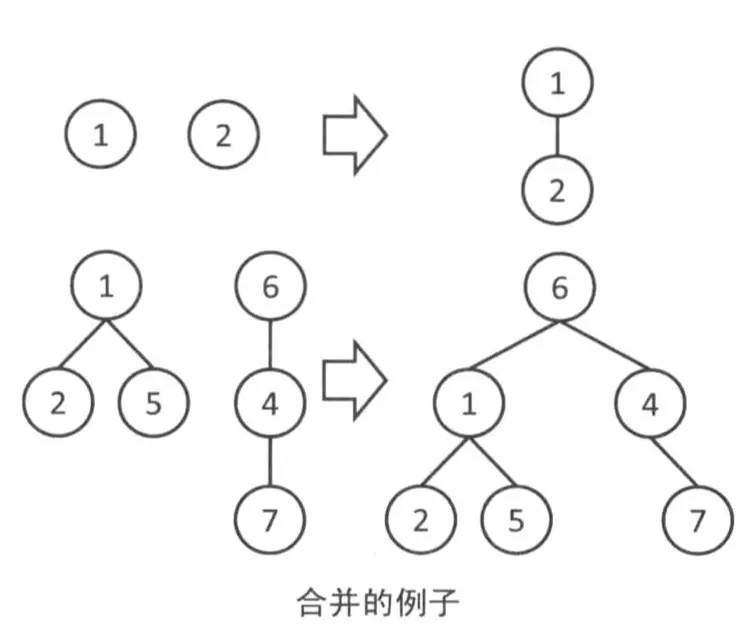void Union(int x,int y){
fa[find(x)]=find(y);
return;
}

1.路径压缩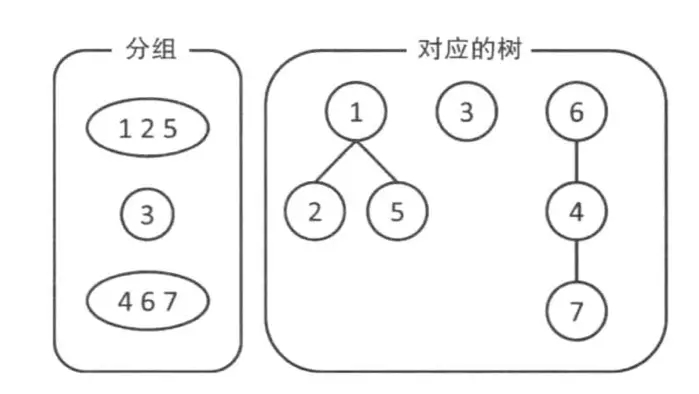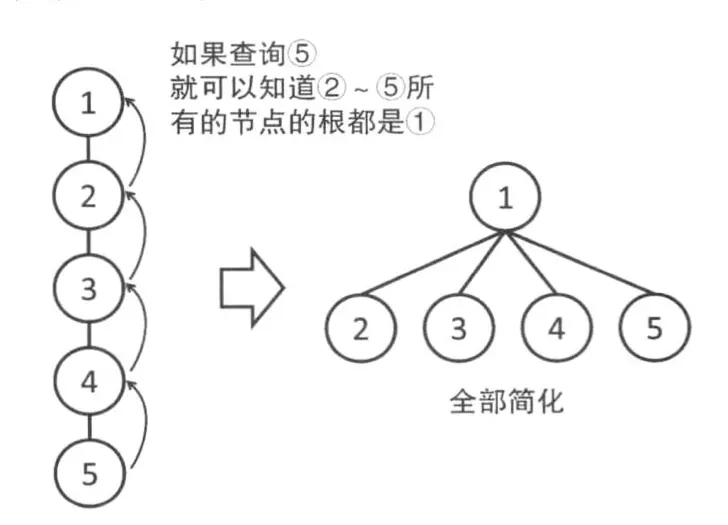int find(int x){
return fa[x]==x?x:fa[x]=find(fa[x]);
}

$\large\text{注意有时候不能使用路径压缩如P2661信息传递}$
2.按秩合并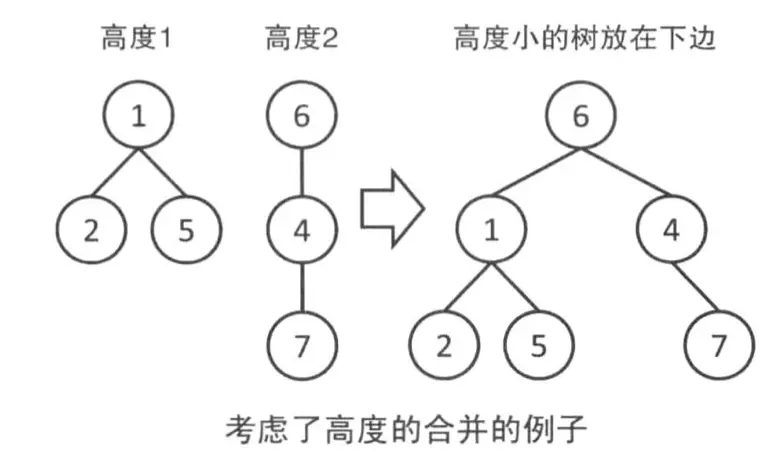void Union(int x,int y){
int rootx=find(x),rooty=find(y);
if(rootx==rooty) return;
if(r[rootx]<r[rooty]) fa[rootx]=rooty;
else if(r[rootx]>r[rooty]) fa[rooty]=rootx;
else{
fa[rootx]=rooty;
r[rooty]++;
}
}

void Union(int x,int y){
int rootx=find(x),rooty=find(y);
if(rootx==rooty) return;
if(size[rootx]>size[rooty]){
fa[rooty]=rootx;
size[rootx]+=size[rooty];
}else{
fa[rootx]=rooty;
size[rooty]+=size[rootx];
}
}

$r$数组用于记录树的高度，$size$数组用于记录树的大小。

posted @ 2019-08-31 16:13 yu__xuan 阅读(...) 评论(...) 编辑 收藏